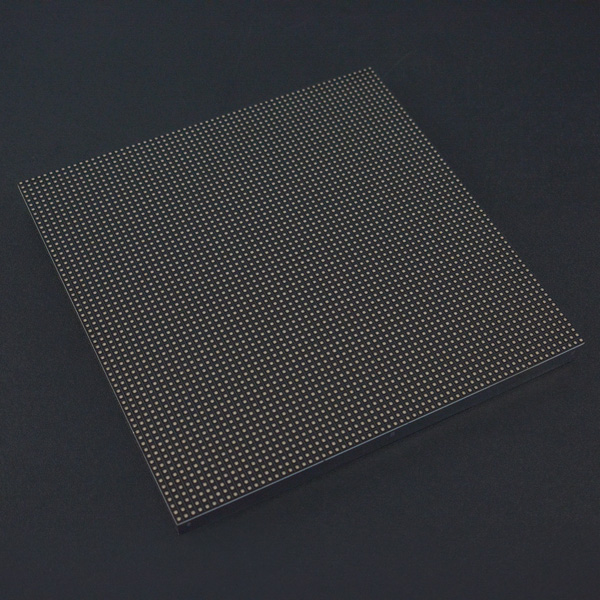## Introduction

City night is always beautiful. She is just like a charming girl, showing her beauty every time. The colorful light is jewelry, dotted on her dress. This is a 64x64 RGB LED Matrix Panel, it has 4096 full-color RGB LEDs in all. Each LED can be independently addressed and controlled. It requires at least 13 digital GPIOs to control the LED matrix. So the UNO board won’t be a good choice in this application, recommended Mega 2560, Raspberry Pi and the other kinds of microcontroller with large RAM and high speed. The led matrix has 2 IDC connectors (DATA_IN, DATA_OUT) on the back, you can cascade multiple panels and make a huge screen together. BUT Arduino doesn’t support this function, its speed is not enough to multiple panel. What's more, it is a high brightness, long life, no pollution, pure color LED display module. It can be used both indoor and outdoor, safety and stability, the module can not be mutually extrusion deformation, also can be used normally in harsh environment.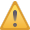Note: This panel needs a 5V@5A power supply.

## Specification

• Operating voltage: DC 5V
• Average power consumption: <500W/㎡
• Maxim Power Consumption: <1000w/㎡
• Pixel: 64x64=4096
• Level of viewing Angle: ≧160°
• Control mode: Synchronous control
• Drive mode: 1/16 scan rate
• Repetition frequency: ≧60Hz
• White Balance Brightness: ≧1200cd/㎡
• Refresh frequency : ≧300Hz
• MTTF: ≧5000 hours
• Service Life: 75000~100000 hours
• Pixel pitch: 3mm
• Dimension: 190 * 190 * 14.5 mm / 7.48 * 7.48 * 0.57 inches
• Thickness: 11mm

### Board Overview

Note: The pin order of DATA-IN and DATA-OUT is same，POWER SUPPLY 5V.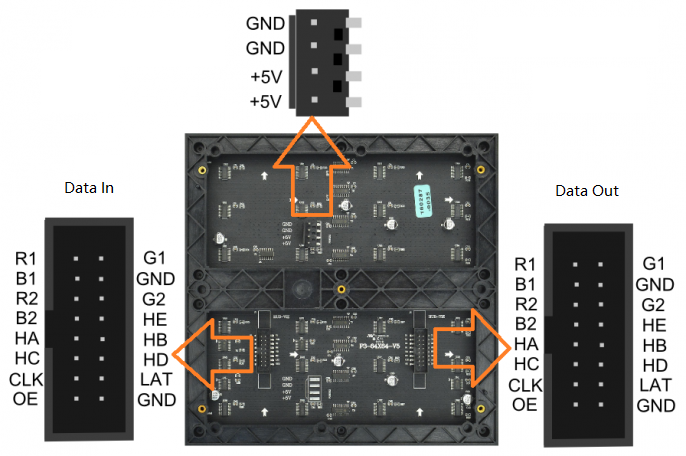Label Name Function
1 DR1 High R data
2 DG1 High G data
3 DB1 High B data
4 GND GND
5 DR2 Low R data
6 DG2 Low G data
7 DB2 Low B data
8 GND GND
9 A A line selection
10 B B line selection
11 C C line selection
12 D D line selection
13 CLK CLOCK
14 LAT LATCH
15 OE Output Enable
16 GND GND

DATA-IN and DATA-OUT

Label Name Function
1 VCC 5V
2 VCC 5V
3 GND GND
4 GND GND

POWER

## Tutorial

According to the pinout to connect, then upload the code to Mega2560, you will be able to see a beautiful display effect.

### Connection Diagram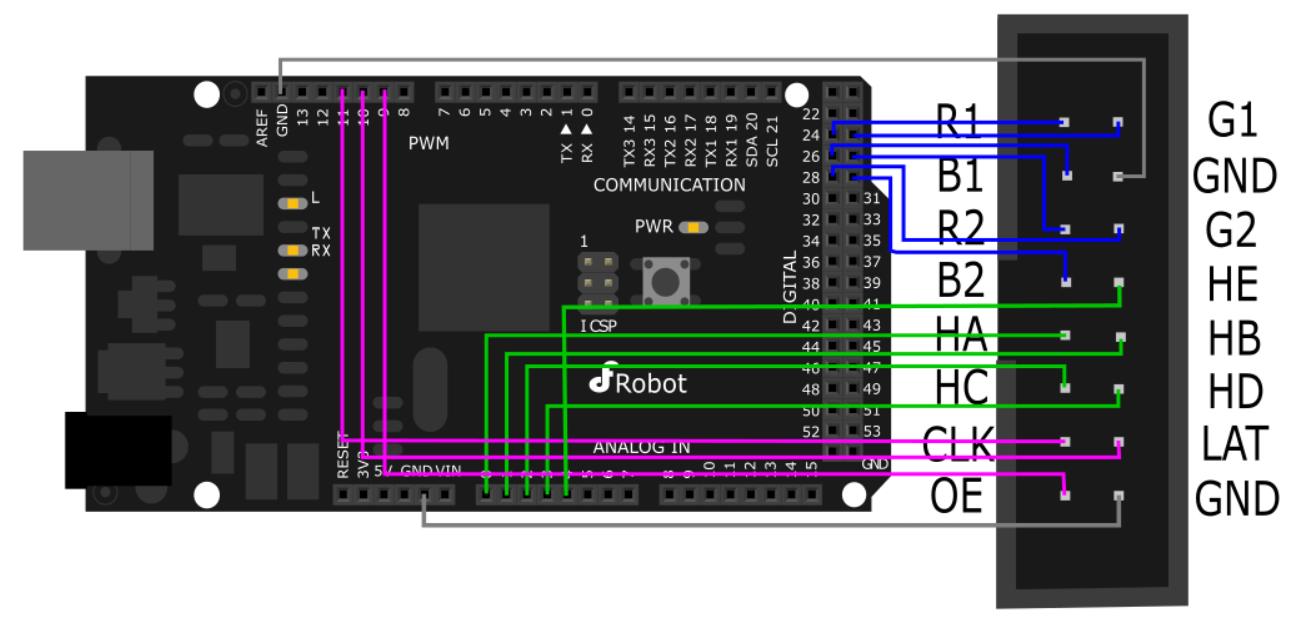Note: It needs an external power supply, the USB is only 5V@500mA, not enough power to drive the screen.

Control signal pin connected to MEGA2560:

R1-> 24 G1-> 25 B1-> 26

R2-> 27 G2-> 28 B2-> 29

HA-> A0 HB-> A1 HC-> A2 HD-> A3 HE-> A4

OE-> 9 LAT-> 10 CLK-> 11

GND-> GND

Power Interface:

-5V -> +5V DC power supply positive

-5V -> +5V DC power supply positive

GND-> DC power supply negative

GND-> DC power supply negative

### 16P Interface Diagram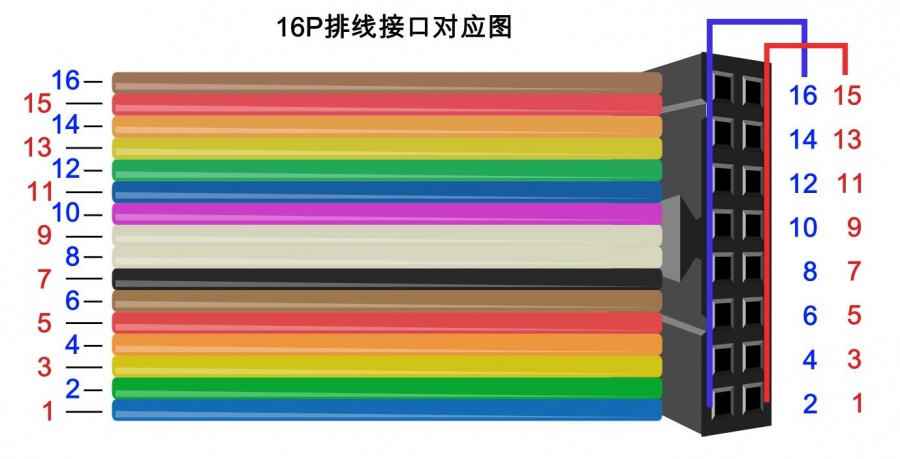### Sample Code 1

``````
#include <DFRobot_RGBMatrix.h> // Hardware-specific library
#include <Wire.h>
#define OE    9
#define LAT   10
#define CLK   11
#define A     A0
#define B     A1
#define C     A2
#define D     A3
#define E     A4
#define WIDTH 64
#define HIGH  64
int a=0;
int b=16;
DFRobot_RGBMatrix matrix(A, B, C, D, E, CLK, LAT, OE, false, WIDTH, HIGH);

void setup()
{
matrix.begin();
// fill the screen with 'black'
matrix.setTextSize(1);     // size 1 == 8 pixels high
matrix.fillScreen(matrix.Color333(0, 0, 0));
delay(500);
}

void loop()
{  byte i;
if(a<=-64)
{a=64;
}
if(b<=-48)
{b=80;
}
matrix.fillScreen(0);//clear the screen
matrix.setTextSize(2);//Set as 16*16 pixel
matrix.setCursor(15, a);    // start at top left, with 8 pixel of spacing
matrix.setTextColor(matrix.Color333(4, 0, 4));//
matrix.println("DFR");//
matrix.setTextSize(1);//Set as 8*8 pixel
matrix.setCursor(12, b);
matrix.println("DFROBOT");
matrix.setTextSize(1);
matrix.print("Let's joinhands witheach otherto create a future");
delay(600);
a=a-6;
b=b-6;
}``````

### Sample Code 2

``````/***************************************************
*
* For 32x32 RGB LED matrix.
*
* @author lg.gang
* @version  V1.0
* @date  2016-10-28
*
* GNU Lesser General Public License.
* All above must be included in any redistribution
* ****************************************************/

#include <Adafruit_GFX.h>   // Core graphics library
#include <RGBmatrixPanel.h> // Hardware-specific library

// use this pinout:
#define CLK 8  // MUST be on PORTB! (Use pin 11 on Mega)
#define OE  9
#define LAT 10
#define A   A0
#define B   A1
#define C   A2
#define D   A3
//#define CLK 8  // MUST be on PORTB! (Use pin 11 on Mega)
//#define LAT 9
//#define OE  10
//#define A   A3
//#define B   A2
//#define C   A1
//#define D   A0
RGBmatrixPanel matrix(A, B, C, D, CLK, LAT, OE, false);

void setup() {
int      x, y, hue;
float    dx, dy, d;
uint8_t  sat, val;
uint16_t c;

matrix.begin();

for(y=0; y < matrix.width(); y++) {
dy = 15.5 - (float)y;
for(x=0; x < matrix.height(); x++) {
dx = 15.5 - (float)x;
d  = dx * dx + dy * dy;
if(d <= (16.5 * 16.5)) { // Inside the circle(ish)?
hue = (int)((atan2(-dy, dx) + PI) * 1536.0 / (PI * 2.0));
d = sqrt(d);
if(d > 15.5) {
// Do a little pseudo anti-aliasing along perimeter
sat = 255;
val = (int)((1.0 - (d - 15.5)) * 255.0 + 0.5);
} else
{
// White at center
sat = (int)(d / 15.5 * 255.0 + 0.5);
val = 255;
}
c = matrix.ColorHSV(hue, sat, val, true);
} else {
c = 0;
}
matrix.drawPixel(x, y, c);
}
}
}

void loop() {
// do nothing
}``````

### Smaple Code 3

``````/***************************************************
*
* For 32x32 RGB LED matrix.
*
* @author lg.gang
* @version  V1.0
* @date  2016-10-28
*
* GNU Lesser General Public License.
* All above must be included in any redistribution
* ****************************************************/

#include <Adafruit_GFX.h>   // Core graphics library
#include <RGBmatrixPanel.h> // Hardware-specific library

// use this pinout:
#define CLK 8  // MUST be on PORTB! (Use pin 11 on Mega)
#define OE  9
#define LAT 10
#define A   A0
#define B   A1
#define C   A2
#define D   A3
//#define CLK 8  // MUST be on PORTB! (Use pin 11 on Mega)
//#define LAT 9
//#define OE  10
//#define A   A3
//#define B   A2
//#define C   A1
//#define D   A0
RGBmatrixPanel matrix(A, B, C, D, CLK, LAT, OE, false);

void setup() {

matrix.begin();

// whew!
}

void loop() {
// fix the screen with red
matrix.fillRect(0, 0, 32, 32, matrix.Color333(7, 0, 0));
delay(500);
// fix the screen with green
matrix.fillRect(0, 0, 32, 32, matrix.Color333(0, 7, 0));
delay(500);
// fix the screen with blue
matrix.fillRect(0, 0, 32, 32, matrix.Color333(0, 0, 7));
delay(500);
// fix the screen with yellow
matrix.fillRect(0, 0, 32, 32, matrix.Color333(7, 7, 0));
delay(500);
// fix the screen with purple
matrix.fillRect(0, 0, 32, 32, matrix.Color333(7, 0, 7));
delay(500);
// fix the screen with cyan
matrix.fillRect(0, 0, 32, 32, matrix.Color333(0,7, 7));
delay(500);
// fix the screen with white
matrix.fillRect(0, 0, 32, 32, matrix.Color333(3, 3, 3));
delay(500);

// fill the screen with 'black'
matrix.fillScreen(matrix.Color333(0, 0, 0));
// draw a pixel in solid white
matrix.drawPixel(0, 0, matrix.Color333(7, 7, 7));
delay(500);

// fix the screen with green
matrix.fillRect(0, 0, 32, 32, matrix.Color333(0, 7, 0));
delay(500);

// draw a box in yellow
matrix.drawRect(0, 0, 32, 32, matrix.Color333(7, 7, 0));
delay(500);

// draw an 'X' in red
matrix.drawLine(0, 0, 31, 31, matrix.Color333(7, 0, 0));
matrix.drawLine(31, 0, 0, 31, matrix.Color333(7, 0, 0));
delay(500);

// draw a blue circle
matrix.drawCircle(10, 10, 10, matrix.Color333(0, 0, 7));
delay(500);

// fill a violet circle
matrix.fillCircle(21, 21, 10, matrix.Color333(7, 0, 7));
delay(500);

// fill the screen with 'black'
matrix.fillScreen(matrix.Color333(0, 0, 0));

// draw some text!
matrix.setCursor(1, 0);    // start at top left, with one pixel of spacing
matrix.setTextSize(1);     // size 1 == 8 pixels high
matrix.setTextWrap(false); // Don't wrap at end of line - will do ourselves

matrix.setTextColor(matrix.Color333(0,7,0));
matrix.println("green");
matrix.setTextColor(matrix.Color333(0,7,7));
matrix.println("robot");

// print each letter with a rainbow color
matrix.setTextColor(matrix.Color333(7,0,0));
matrix.print('3');
matrix.setTextColor(matrix.Color333(7,4,0));
matrix.print('2');
matrix.setTextColor(matrix.Color333(7,7,0));
matrix.print('x');
matrix.setTextColor(matrix.Color333(4,7,0));
matrix.print('3');
matrix.setTextColor(matrix.Color333(0,7,0));
matrix.println('2');

matrix.setTextColor(matrix.Color333(0,7,7));
matrix.print('*');
matrix.setTextColor(matrix.Color333(0,4,7));
matrix.print('R');
matrix.setTextColor(matrix.Color333(0,0,7));
matrix.print('G');
matrix.setTextColor(matrix.Color333(4,0,7));
matrix.print('B');
matrix.setTextColor(matrix.Color333(7,0,4));
matrix.print('*');
delay(5000);
}``````

### Expected Results

• Sample Code 1: Character Display
• Sample Code 2: A color filled circle
• Sample Code 3: The LED module will take turns display: a white point, full screen green, full screen yellow, a yellow rectangle, a yellow rectangle, a red X and a blue circle,

## FAQ

For any questions, advice or cool ideas to share, please visit the DFRobot Forum.

## More Documents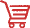Shopping from 32x32 RGB LED Matrix Panel (4mm pitch) or DFRobot Distributor.

Turn to the Top